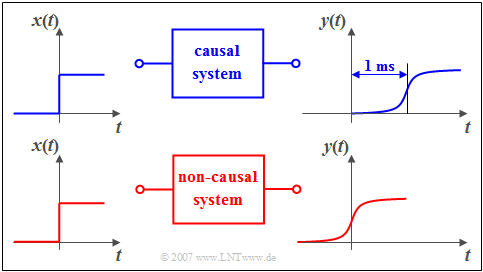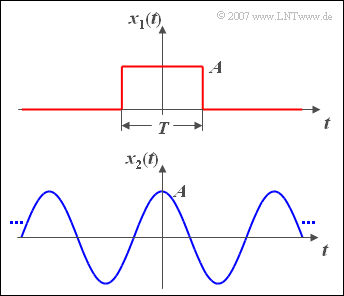# Signal#

## Notation#

Name Description
Time function $$x(t)$$
Spectral function $$X(f)$$
Sampling Time $$T_A = \frac{1}{f_A}$$
Sampling Frequency $$f_A = \frac{1}{T_A}$$
Symbol Notation.

### Rectangular Pulse#

mpl{name=rectangle} fig, ax = plt.subplots() N = 100 # sample count P = 10 # period D = 5 # width of pulse sig = np.arange(N) % P < D ax.plot(sig)

## Signal Categories#

Details:

If a signal is both value--continuous and time--continuous, it is called a analog signal. A digital signal, on the other hand, is always value--discrete and time-discrete and the message contained therein consists of the symbols of a symbol set.

• Time-Continuous Signal: the signal parameter $$t$$ is defined at any given time.
• Time-Discrete Signal: the signal parameter is defined only at the discrete points $$t_v = v \cdot T_A$$.
• Deterministic Signal: the time function $$x(t)$$ can be described completely in analytical form.
• Random Signal: its time function $$x(t)$$ is not -- or at least not completely -- describable in mathematical form. Such a signal cannot be predicted exactly for the future.
• Causal Signal/System: if $$x(t)$$ does not exist for all times $$t < 0$$ or is identical zero.
• Energy--Limited Signal: if $$x(t)$$ has finite energy $$E_x$$ and infinitely small power ($$P_x \to 0$$)
• Power--Limited Signal: if $$x(t)$$ has finite power $$P_x$$ and infinite energy $$(E_x \to \infty)$$.
Examples of SignalsYou can see a causal system in the upper graphic: If a unit step function $$x(t)$$ is applied to its input, then the output signal 𝑦(𝑡) can only increase from zero to its maximum value after time 𝑡=0. In the lower graph the causality is no longer given. As you can easily see in this example, an additional runtime of one millisecond is enough to change from the non-causal to the causal representation.

## Energy--Limited and Power--Limited Signals#

Both, energy and power of a signal, are dependent on the resistance 𝑅. In order to eliminate this dependency, the resistance 𝑅=1Ω is often used as a basis in communications engineering. Then the following definitions apply:

The energy in $$\si{\text{V}^2\text{s}}$$ of the signal $$x(t)$$ is:

$E_x=\lim_{T_{\rm M}\to\infty} \int^{T_{\rm M}/2} _{-T_{\rm M}/2} x^2(t)\,{\rm d}t.$

with $$T_M$$ is the assumed measurement duration during which the signal is observed, symmetrically with respect to the time origin ($$t=0$$). In general, this time interval must be chosen very large; ideally $$T_M$$ should be towards infinity.

The (mean) power in $$\si{\text{V}^2}$$ is the energy divided by the time before the limit crossing:

$P_x = \lim_{T_{\rm M} \to \infty} \frac{1}{T_{\rm M} } \cdot \int^{T_{\rm M}/2} _{-T_{\rm M}/2} x^2(t)\,{\rm d}t.$

with $$T_M$$ is the assumed measurement duration during which the signal is observed, symmetrically with respect to the time origin ($$t=0$$). In general, this time interval must be chosen very large; ideally $$T_M$$ should be towards infinity.

Example Energy and Power Calculations

Now the energy and power of two exemplary signals are calculated.The upper graphic shows a rectangular pulse $$x_1(t)$$ with amplitude $$A$$ and duration $$T$$.

• The signal energy of this pulse is $$E_1 = A^2 \cdot T$$.
• For the signal power, division by $$T_{\rm M}$$ and limit formation $$(T_{\rm M} \to \infty)$$ the value is $$P_1 = 0$$.

For the cosine signal $$x_2(t)$$ with amplitude $$A$$ applies according to the sketch below:

• The signal power is $$P_2 = A^2/2$$, regardless of the frequency.
• The signal energy $$E_2$$ (integral over power for all times) is infinite.

## Signals and Spectrum#

Time $$x(t)$$ Frequency $$X(f)$$